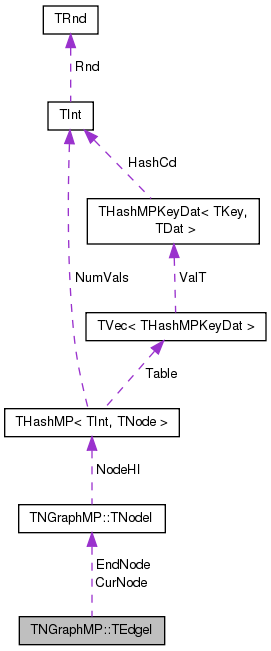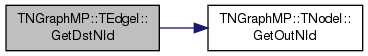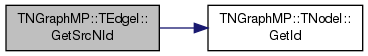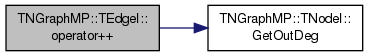SNAP Library 6.0, Developer Reference  2020-12-09 16:24:20 SNAP, a general purpose, high performance system for analysis and manipulation of large networks
TNGraphMP::TEdgeI Class Reference

Edge iterator. Only forward iteration (operator++) is supported. More...

`#include <graphmp.h>`

Collaboration diagram for TNGraphMP::TEdgeI:[legend]

## Public Member Functions

TEdgeI ()

TEdgeI (const TNodeI &NodeI, const TNodeI &EndNodeI, const int &EdgeN=0)

TEdgeI (const TEdgeI &EdgeI)

TEdgeIoperator= (const TEdgeI &EdgeI)

TEdgeIoperator++ (int)
Increment iterator. More...

bool operator< (const TEdgeI &EdgeI) const

bool operator== (const TEdgeI &EdgeI) const

int GetId () const
Gets edge ID. Always returns -1 since only edges in multigraphs have explicit IDs. More...

int GetSrcNId () const
Gets the source node of an edge. More...

int GetDstNId () const
Gets destination node of an edge. More...

TNodeI CurNode

TNodeI EndNode

int CurEdge

class TNGraphMP

## Detailed Description

Edge iterator. Only forward iteration (operator++) is supported.

Definition at line 102 of file graphmp.h.

## Constructor & Destructor Documentation

 TNGraphMP::TEdgeI::TEdgeI ( )
inline

Definition at line 107 of file graphmp.h.

107 : CurNode(), EndNode(), CurEdge(0) { }
TNodeI CurNode
Definition: graphmp.h:104
TNodeI EndNode
Definition: graphmp.h:104
 TNGraphMP::TEdgeI::TEdgeI ( const TNodeI & NodeI, const TNodeI & EndNodeI, const int & EdgeN = `0` )
inline

Definition at line 108 of file graphmp.h.

108 : CurNode(NodeI), EndNode(EndNodeI), CurEdge(EdgeN) { }
TNodeI CurNode
Definition: graphmp.h:104
TNodeI EndNode
Definition: graphmp.h:104
 TNGraphMP::TEdgeI::TEdgeI ( const TEdgeI & EdgeI )
inline

Definition at line 109 of file graphmp.h.

109 : CurNode(EdgeI.CurNode), EndNode(EdgeI.EndNode), CurEdge(EdgeI.CurEdge) { }
TNodeI CurNode
Definition: graphmp.h:104
TNodeI EndNode
Definition: graphmp.h:104

## Member Function Documentation

 int TNGraphMP::TEdgeI::GetDstNId ( ) const
inline

Gets destination node of an edge.

Definition at line 121 of file graphmp.h.

References TNGraphMP::TNodeI::GetOutNId().

121 { return CurNode.GetOutNId(CurEdge); }
int GetOutNId(const int &NodeN) const
Returns ID of NodeN-th out-node (the node the current node points to).
Definition: graphmp.h:88
TNodeI CurNode
Definition: graphmp.h:104

Here is the call graph for this function:Here is the caller graph for this function:int TNGraphMP::TEdgeI::GetId ( ) const
inline

Gets edge ID. Always returns -1 since only edges in multigraphs have explicit IDs.

Definition at line 117 of file graphmp.h.

117 { return -1; }
 int TNGraphMP::TEdgeI::GetSrcNId ( ) const
inline

Gets the source node of an edge.

Definition at line 119 of file graphmp.h.

References TNGraphMP::TNodeI::GetId().

119 { return CurNode.GetId(); }
int GetId() const
Returns ID of the current node.
Definition: graphmp.h:72
TNodeI CurNode
Definition: graphmp.h:104

Here is the call graph for this function:Here is the caller graph for this function:TEdgeI& TNGraphMP::TEdgeI::operator++ ( int )
inline

Increment iterator.

Definition at line 112 of file graphmp.h.

References TNGraphMP::TNodeI::GetOutDeg().

112  { CurEdge++; if (CurEdge >= CurNode.GetOutDeg()) { CurEdge=0; CurNode++;
113  while (CurNode < EndNode && CurNode.GetOutDeg()==0) { CurNode++; } } return *this; }
int GetOutDeg() const
Returns out-degree of the current node.
Definition: graphmp.h:78
TNodeI CurNode
Definition: graphmp.h:104
TNodeI EndNode
Definition: graphmp.h:104

Here is the call graph for this function:bool TNGraphMP::TEdgeI::operator< ( const TEdgeI & EdgeI ) const
inline

Definition at line 114 of file graphmp.h.

References CurEdge, and CurNode.

114 { return CurNode<EdgeI.CurNode || (CurNode==EdgeI.CurNode && CurEdge<EdgeI.CurEdge); }
TNodeI CurNode
Definition: graphmp.h:104
 TEdgeI& TNGraphMP::TEdgeI::operator= ( const TEdgeI & EdgeI )
inline

Definition at line 110 of file graphmp.h.

References CurEdge, CurNode, and EndNode.

110 { if (this!=&EdgeI) { CurNode=EdgeI.CurNode; EndNode=EdgeI.EndNode; CurEdge=EdgeI.CurEdge; } return *this; }
TNodeI CurNode
Definition: graphmp.h:104
TNodeI EndNode
Definition: graphmp.h:104
 bool TNGraphMP::TEdgeI::operator== ( const TEdgeI & EdgeI ) const
inline

Definition at line 115 of file graphmp.h.

References CurEdge, and CurNode.

115 { return CurNode == EdgeI.CurNode && CurEdge == EdgeI.CurEdge; }
TNodeI CurNode
Definition: graphmp.h:104

## Friends And Related Function Documentation

 friend class TNGraphMP
friend

Definition at line 122 of file graphmp.h.

## Member Data Documentation

 int TNGraphMP::TEdgeI::CurEdge
private

Definition at line 105 of file graphmp.h.

Referenced by operator<(), operator=(), and operator==().

 TNodeI TNGraphMP::TEdgeI::CurNode
private

Definition at line 104 of file graphmp.h.

Referenced by operator<(), operator=(), and operator==().

 TNodeI TNGraphMP::TEdgeI::EndNode
private

Definition at line 104 of file graphmp.h.

Referenced by operator=().

The documentation for this class was generated from the following file: